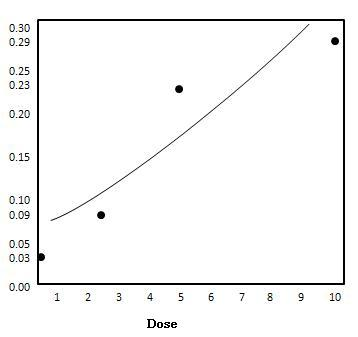# Statistics - Logistic Regression

Logistic regression is a statistical method for analyzing a dataset in which there are one or more independent variables that determine an outcome. The outcome is measured with a dichotomous variable (in which there are only two possible outcomes).

## Formula

${\pi(x) = \frac{e^{\alpha + \beta x}}{1 + e^{\alpha + \beta x}}}$

Where −

• Response - Presence/Absence of characteristic.

• Predictor - Numeric variable observed for each case

• ${\beta = 0 \Rightarrow }$ P (Presence) is the same at each level of x.

• ${\beta \gt 0 \Rightarrow }$ P (Presence) increases as x increases

• ${\beta = 0 \Rightarrow }$ P (Presence) decreases as x increases.

### Example

Problem Statement:

Solve the logistic regression of the following problem Rizatriptan for Migraine

Response - Complete Pain Relief at 2 hours (Yes/No).

Predictor - Dose (mg): Placebo (0), 2.5,5,10

Dose#Patients#Relieved%Relieved
06723.0
2.57579.3
51302922.3
101454027.6

Solution:

Having ${\alpha = -2.490} and${\beta = .165}, we've following data:

${\pi(0) = \frac{e^{\alpha + \beta \times 0}}{1 + e^{\alpha + \beta \times 0}} \\[7pt] \, = \frac{e^{-2.490 + 0}}{1 + e^{-2.490}} \\[7pt] \\[7pt] \, = 0.03 \\[7pt] \pi(2.5) = \frac{e^{\alpha + \beta \times 2.5}}{1 + e^{\alpha + \beta \times 2.5}} \\[7pt] \, = \frac{e^{-2.490 + .165 \times 2.5}}{1 + e^{-2.490 + .165 \times 2.5}} \\[7pt] \, = 0.09 \\[7pt] \\[7pt] \pi(5) = \frac{e^{\alpha + \beta \times 5}}{1 + e^{\alpha + \beta \times 5}} \\[7pt] \, = \frac{e^{-2.490 + .165 \times 5}}{1 + e^{-2.490 + .165 \times 5}} \\[7pt] \, = 0.23 \\[7pt] \\[7pt] \pi(10) = \frac{e^{\alpha + \beta \times 10}}{1 + e^{\alpha + \beta \times 10}} \\[7pt] \, = \frac{e^{-2.490 + .165 \times 10}}{1 + e^{-2.490 + .165 \times 10}} \\[7pt] \, = 0.29 }$
Dose(${x}$)${\pi(x)}$
00.03
2.50.09
50.23
100.29